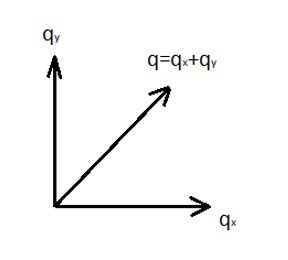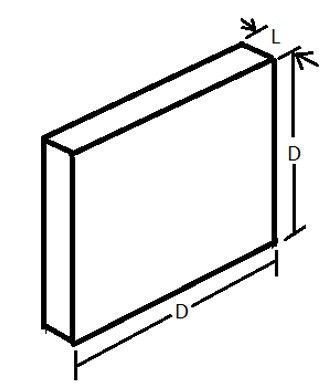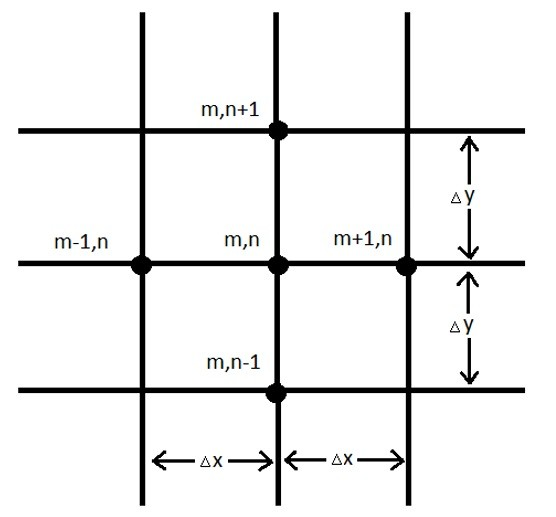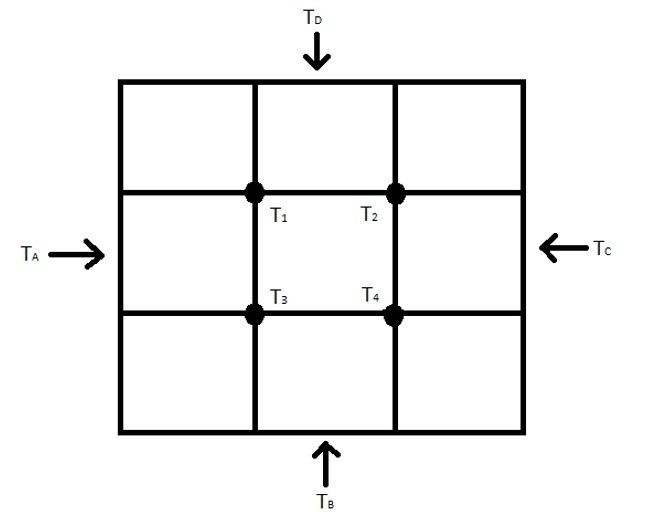There are different methods that can be used to determine heat transfer in multiple directions. Some methods are more complicated than others. I will be talking about three methods that could be used. They are the mathematical analysis, the conduction shape factor, or numerical methods of analysis.

## Mathematical Analysis

First let’s briefly go over the math behind a multiple dimensional problem. To calculate heat transfer in multiple directions the Laplace equation would be used. Refer to the equation below for a two dimensional problem.

(Eq 1)  $\frac{∂^2T}{∂x^2} + \frac{∂^2T}{∂y^2} = 0$

Equation 1 will provide the temperature in a two-dimensional body as a function of the two spatial coordinates x and y for a steady state problem. This will result in the following Fourier equations.

(Eq2)  $q_x = -kA_x\frac{∂T}{∂x}$

(Eq3)  $q_y = -kA_y\frac{∂T}{∂y}$

This means that the heat flow through a two dimensional problem is a resultant of the heat flow in both the x and y direction as seen in the image below.To solve equation 1 the separation-of-variable method would be used. This is done because it is assumed that the solution to the differential equations will take a product form as seen in the equation below.

(Eq 4)  $T = XY$

where X = X(x) and Y = Y(y)

Boundary conditions must then be applied to determine the form of the functions X and Y.

Finally, inputting equation 4 into equation 1 will than result in the following equation.

(Eq 5)  $-\frac{1}{X} \frac{d^2X}{dx^2} = \frac{1}{Y} \frac{d^2Y}{dy^2}$

Since X and Y are independent variables, equation 5 can be broken down into two separate differential equations.

(Eq 6) $\frac{d^2X}{dx^2}+λ^2X = 0$

(Eq 7) $\frac{d^2Y}{dx^2}+λ^2Y = 0$

Where λ2 represent the separation constant. Its value will then be determine by the boundary conditions of the problem.

The following tutorial provides some excellent examples on how to solve these types of differential equations. Also, be aware, depending on you boundary conditions these types of problem can become very complex. If you have the option, using an FEA program would be the better option for a problem that has complex boundary conditions.

## Conduction Shape Factor

Above I discussed how the mathematical analysis could be used to solve for the heat transfer through a two-dimensional object. However, as mentioned this can become a complex process where you need to have a firm understand of differential equations. A simpler method to solve the total heat transfer through a multiple dimension object would be to use a predefined conduction shape factor. If you have a predetermined conduction shape factor than the equation below would be used.

(Eq 8) $q = kSΔT_{overall}$

The variable S in the equation above represents the conduction shape factor.

For example if you are trying to calculate the heat transfer rate through a three-dimensional wall the following conduction shape factors would be used.

(Eq 9) $S_{wall} = \frac{A}{L}$

(Eq 10) $S_{edge} = 0.54D$

(Eq 11)  $S_{corner} = 0.15L$The above equations can only be used when the interior dimensions of the wall are greater than one-fifth that of the total wall thickness.

For the example, what if you had a small cubical furnace that is 1m X 1m X 1m with a wall thickness of 0.1 m. The inside of the furnace is maintained at 450 degrees C, while the temperature on the outside of the furnace is 20 degrees C. The thermal conductivity of the furnace walls is .75 W/m-C. How much heat is lost through the walls of the furnace?

The first thing that we need to realize is that there are six wall sections, which means there are twelve edges, and eight corners. This will result in the following conduction shape factor.

(Eq 12) $S = \frac{6A}{L} + 12(0.54D) + 8(0.15L)$

(Eq 13)  $S = 6\left(\frac{1}{0.1}\right) +12 (0.54)(1) + 8(0.15)(0.1)$

Now that the conduction shape factor has been found the total heat loss through the walls of the furnace can be determined using equation 8.

(Eq 14) $q = (.75)(66.6)(450-20) = 21.5kW$

Different conductive shape factors can be found in various heat transfer text books.

## Numerical Method of Analysis

Another method that can be used that is much less complex than the mathematical method is called the numerical method of analysis which is also known as the finite – difference technique. To use this method the object of interest would be divided into equal increments known as a mesh. Where the lines of the mesh intersect a node point will be created. This can be seen in the image below.At the node points for a 2 dimensional problem, the m locations represent the x increments while the n locations represent the y increments. Finite differences will then be used to determine the temperature at each node point. The resultant temperatures will be approximations of the actual temperature. Due to this fact, the finer the mesh the closer the results will be. The following equation would be used to determine the temperature at node point m,n.

(Eq 15) $T_{m+1,n} + T_{m-1,n} + T_{m,n+1} + T_{m,n-1} – 4T_{m,n} = 0$

The above equation would then be applied to the remaining nodes and the temperature at each node point could then be solved algebraically. Refer to the example below.The equations below represent the temperatures at nodes 1, 2, 3, and 4 for the image above.

(Eq 16) $T_A + T_D + T_2 + T_3 – 4T_1 = 0$

(Eq 17) $T_C + T_D + T_1 + T_4 – 4T_2 = 0$

(Eq 18) $T_A + T_B + T_1 + T_4 – 4T_3 = 0$

(Eq 19) $T_B + T_C + T_2 + T_3 – 4T_4 = 0$

Once the temperature values at the nodes are determined the total heat transfer through a specific face can be determined using the following equation.

(Eq 20)  $q = ∑kΔx\frac{ΔT}{Δy}$

Finally, let’s say for the above example that TA is the highest temperature out of the four. This means the heat will transfer from TA, to TB, TC, and TD. The total heat flow would be represented by the follow to equations.

(Eq 21) $q = -k\frac{Δx}{Δy}[(T_1-T_A) + (T_3 – T_A)]$

Or

(Eq 22) $q = -k\frac{Δy}{Δx}[(T_3 – T_B) + (T_4 – T_B) + (T_4 – T_C) + (T_2 – T_C) + (T_1 – T_D) + (T_2 – T_D)]$